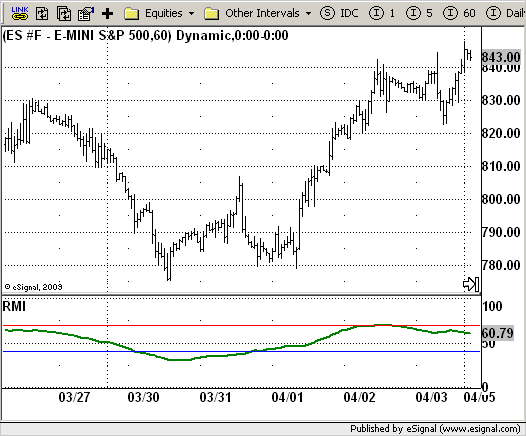# Relative Momentum Index (RMI)

ICE Data Services -

RMI.efs

File Name: RMI.efs

Description:
Relative Momentum Index (RMI)

Formula Parameters:

• Length : 20
• SellZone : 70
• Source of Price : Close

Notes:
The Relative Momentum Index (RMI) was developed by Roger Altman. Impressed with the Relative Strength Index's sensitivity to the number of look-back periods, yet frustrated with it's inconsistent oscillation between defined overbought and oversold levels, Mr. Altman added a momentum component to the RSI.
As mentioned, the RMI is a variation of the RSI indicator. Instead of counting up and down days from close to close as the RSI does, the RMI counts up and down days from the close relative to the close x-days ago where x is not necessarily 1 as required by the RSI). So as the name of the indicator reflects, "momentum" is substituted for "strength".

RMI.efsEFS Code:

```/*********************************
Provided By:
eSignal (Copyright c eSignal), a division of Interactive Data
Formula Script (EFS) is for educational purposes only and may be
modified and saved under a new file name.  eSignal is not responsible
for the functionality once modified.  eSignal reserves the right
to modify and overwrite this EFS file with each new release.

Description:
Relative Momentum Index (RMI)

Version:            1.0  04/29/2009

Formula Parameters:                     Default:
Length                              20
SellZone                            70
Source of Price                     Close

Notes:
The Relative Momentum Index (RMI) was developed by Roger Altman. Impressed
with the Relative Strength Index's sensitivity to the number of look-back
periods, yet frustrated with it's inconsistent oscillation between defined
overbought and oversold levels, Mr. Altman added a momentum component to the RSI.
As mentioned, the RMI is a variation of the RSI indicator. Instead of counting
up and down days from close to close as the RSI does, the RMI counts up and down
days from the close relative to the close x-days ago where x is not necessarily
1 as required by the RSI). So as the name of the indicator reflects, "momentum" is
substituted for "strength".

**********************************/
var fpArray = new Array();
var bInit = false;

function preMain() {
setPriceStudy(false);
setStudyTitle("RMI");
setCursorLabelName("RMI", 0);
setShowTitleParameters(false);
setDefaultBarFgColor(Color.green, 0);
setDefaultBarThickness(2, 0);
setStudyMax(101);
setStudyMin(-1);
var x = 0;
fpArray[x] = new FunctionParameter("Length", FunctionParameter.NUMBER);
with(fpArray[x++]) {
setLowerLimit(1);
setDefault(20);
}
with(fpArray[x++]) {
setLowerLimit(1);
setDefault(40);
}
fpArray[x] = new FunctionParameter("SellZone", FunctionParameter.NUMBER);
with(fpArray[x++]) {
setLowerLimit(1);
setDefault(70);
}
fpArray[x] = new FunctionParameter("sPrice", FunctionParameter.STRING);
with(fpArray[x++]){
setName("Source of Price");
setDefault("close");
}
}

var xRMI = null;

function main(sPrice, Length, BuyZone, SellZone) {
var nBarState = getBarState();
var nRMI = 0;
if (nBarState == BARSTATE_ALLBARS) {
if (sPrice == null) sPrice = "close";
if (Length == null) Length = 20;
if (SellZone == null) SellZone = 70;
}
if (bInit == false) {
xRMI = efsInternal("Calc_RMI", sPrice, Length)
bInit = true;
}
nRMI = xRMI.getValue(0);
if (nRMI == null) return;
return nRMI;
}

var bSecondInit = false;
var xMU = null;
var xMD = null;
var xMOM = null;

function Calc_RMI(sPrice, Length) {
var Momentum = 0;
var nMU = 0;
var nMD = 0;
if (getCurrentBarCount() <= Length) return;
if (bSecondInit == false) {
xMOM =  mom(Length, eval(sPrice)());
xMU = efsInternal("Calc_MU", Length, xMOM);
xMD = efsInternal("Calc_MD", Length, xMOM);
bSecondInit = true;
}
nMU = xMU.getValue(0);
nMD = xMD.getValue(0);
if (nMU == null || nMD == null) return;
var RM = nMU / nMD;
var RMI = 100 * (RM / (1 + RM));
return RMI;
}

function  Calc_MU(Length, xSeries) {
var nRes = null;
var nRef = ref(-1);
var Momentum = xSeries.getValue(0);
if (xSeries.getValue(-1) == null) return;
if (Momentum >= 0) nRes = nRef - (nRef / Length) + Momentum
else nRes = nRef;
if (nRes == null) return;
return nRes;
}

function  Calc_MD(Length, xSeries) {
var nRes = null;
var nRef = ref(-1);
var Momentum = xSeries.getValue(0);
if (xSeries.getValue(-1) == null) return;
if (Momentum <= 0) nRes = nRef - (nRef / Length) + Math.abs(Momentum)
else nRes = nRef;
if (nRes == null) return;
return nRes;
}```